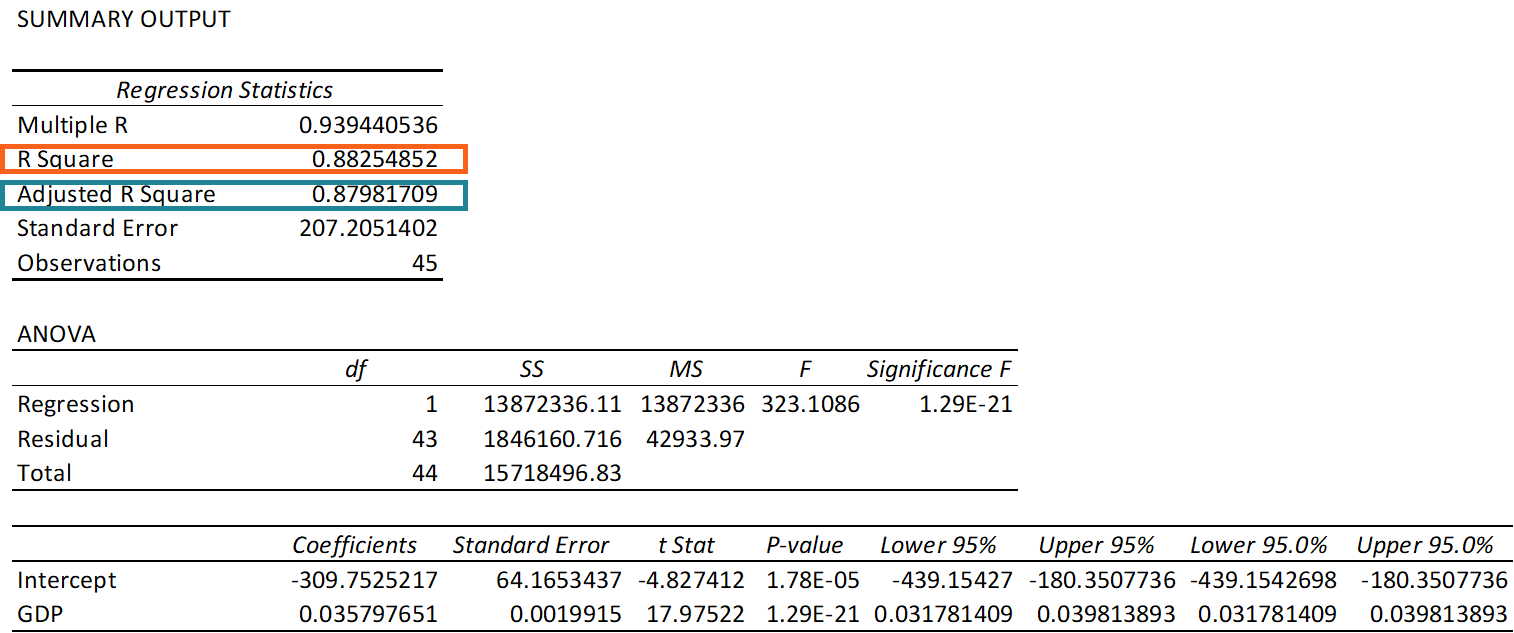# Coefficient of Determination

A statistical measure that determines the proportion of variance in the dependent variable that can be explained by the independent variable

## What is the Coefficient of Determination?

The coefficient of determination (R² or r-squared) is a statistical measure in a regression model that determines the proportion of variance in the dependent variable that can be explained by the independent variable. In other words, the coefficient of determination tells one how well the data fits the model (the goodness of fit).Although the coefficient of determination provides some useful insights regarding the regression model, one should not rely solely on the measure in the assessment of a statistical model. It does not disclose information about the causation relationship between the independent and dependent variables as well as it does not indicate the correctness of the regression model. Therefore, the user should always draw conclusions about the model by analyzing the coefficient of determination together with other variables in a statistical model.

The coefficient of determination can take any values between 0 to 1. In addition, the statistical metric is frequently expressed in percentages.

### Interpretation of the Coefficient of Determination (R²)

The most common interpretation of the coefficient of determination is how well the regression model fits the observed data. For example, a coefficient of determination of 60% shows that 60% of the data fit the regression model. Generally, a higher coefficient indicates a better fit for the model.

However, it is not always the case that a high r-squared is good for the regression model. The quality of the coefficient of determination depends on several factors, including the units of measure of the variables, the nature of the variables employed in the model, and the applied data transformation. Thus, sometimes, a high coefficient of determination can indicate issues with the regression model.

No universal rule governs how to incorporate the coefficient of determination in the assessment of a model. The context in which the forecast or the experiment is based is extremely important, and in different scenarios, the insights from the statistical metric can vary.

### Calculation of the Coefficient of Determination (R²)

Mathematically, the coefficient of determination can be found using the following formula:Where:

• SSregression – the sum of squares due to regression (explained sum of squares)
• SStotal –  the total sum of squares

Although the terms “total sum of squares” and “sum of squares due to regression” seem confusing, the variables’ meanings are straightforward.

The total sum of squares measures the variation in the observed data (data used in regression modeling). The sum of squares due to regression measures how well the regression model represents the data that were used for modeling.

### More Resources

CFI is the official provider of the global Financial Modeling & Valuation Analyst (FMVA)™ certification program, designed to help anyone become a world-class financial analyst. To keep advancing your career, the additional resources below will be useful:

• Basic Statistics Concepts in Finance
• Binomial Distribution
• Central Limit Theorem
• Regression Analysis

### Financial Analyst Certification

Become a certified Financial Modeling and Valuation Analyst (FMVA)® by completing CFI’s online financial modeling classes and training program!